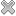Courses / Module

Toggle PrintGENERAL CHEMISTRY

Module code: CH101P
Credits: 7.5
Semester: 1
Department: CHEMISTRY
International:Overview

Introduction and stoichiometry; Chemistry in aqueous solutions; Atoms, molecules and the Periodic Table; Atomic structure and shapes of atomic orbitals; Bond formation, ionic and covalent bonds; Lewis structures, VSEPR theory and geometry predictions for polyatomic molecules; Physical trends in the Periodic Table.

This module covers four important areas in Chemistry:

(i) Introduction to Chemistry: Elements and their physical characteristics; Introduction to Atomic Theory - physical mixtures versus chemical compounds; Covalent and Ionic bonding, the naming of chemical compounds, expression of chemical reactions; The mole concept; The chemical equation; Relative atomic mass; Stoichiometric calculations and balancing chemical equations using mole quantities; The ideal gas equation and units; Empirical and molecular formulae of compounds from elemental analysis; The determination of relative atomic mass from chemical reaction data; Solutions, concentration, molarity; Calculations using titrimetric data; Stoichiometric calculations for reactions involving solid, dissolved and gaseous reagents. Classification of common inorganic reactions.

(ii) Chemistry in Solution - Acid and Base Reactions: Bronsted-Lowry theory of acids and bases; Strong and weak acids; pH of strong and weak acids/bases; Titration curves; Indicators; Buffer solutions; pH of salt solutions; Redox reactions - Concept of oxidation state and reactions involving a change in oxidation state.

(iii) Atoms, Molecules and the Periodic Table. The topics covered in this section are: Historical development of atomic structure; Bohr theory; Ionization potentials; Atomic quantum number; Aufbau principle; Electronic basis of the Periodic Table; Groups, periods and blocks; Basic chemistry of the elements based on the electronic structures of atoms; Electronegativities; Wave mechanical theory of the H-atom; Schrodinger''s equation (angular and radial functions, radial distribution functions); Shapes of orbitals; Pauli principle, electron spin and Hund's rules; Simple concepts of chemical bond formation (ionic/covalent bonds); Lewis model; Valence shell electron-pair repulsion (VSEPR) theory; Prediction of geometries for polyatomic molecules.

(iv) Physical Trends in the Periodic Table. Atomic and ionic sizes, ionisation energy, electron affinity, electronegativity; Variation in the type of compound formed across the table - some relationships between position on the periodic table and bond strength.Learning OutcomesTeaching & Learning methodsAssessmentRepeat optionsTimetable